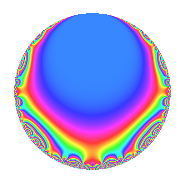# Properties

 Label 731.2.a.bLevel 731 Weight 2 Character orbit 731.a Self dual Yes Analytic conductor 5.837 Analytic rank 1 Dimension 2 CM No Inner twists 1

# Related objects

## Newspace parameters

 Level: $$N$$ = $$731 = 17 \cdot 43$$ Weight: $$k$$ = $$2$$ Character orbit: $$[\chi]$$ = 731.a (trivial)

## Newform invariants

 Self dual: Yes Analytic conductor: $$5.83706438776$$ Analytic rank: $$1$$ Dimension: $$2$$ Coefficient field: $$\Q(\sqrt{17})$$ Coefficient ring: $$\Z[a_1, a_2, a_3]$$ Coefficient ring index: $$1$$ Fricke sign: $$1$$ Sato-Tate group: $\mathrm{SU}(2)$

## $q$-expansion

Coefficients of the $$q$$-expansion are expressed in terms of $$\beta = \frac{1}{2}(1 + \sqrt{17})$$. We also show the integral $$q$$-expansion of the trace form.

 $$f(q)$$ $$=$$ $$q - q^{2} + ( 1 - \beta ) q^{3} - q^{4} + ( -1 + \beta ) q^{5} + ( -1 + \beta ) q^{6} + 3 q^{8} + ( 2 - \beta ) q^{9} +O(q^{10})$$ $$q - q^{2} + ( 1 - \beta ) q^{3} - q^{4} + ( -1 + \beta ) q^{5} + ( -1 + \beta ) q^{6} + 3 q^{8} + ( 2 - \beta ) q^{9} + ( 1 - \beta ) q^{10} -2 q^{11} + ( -1 + \beta ) q^{12} + ( -3 + \beta ) q^{13} + ( -5 + \beta ) q^{15} - q^{16} - q^{17} + ( -2 + \beta ) q^{18} + ( 2 + 2 \beta ) q^{19} + ( 1 - \beta ) q^{20} + 2 q^{22} + 2 q^{23} + ( 3 - 3 \beta ) q^{24} -\beta q^{25} + ( 3 - \beta ) q^{26} + ( 3 + \beta ) q^{27} + ( -2 + 2 \beta ) q^{29} + ( 5 - \beta ) q^{30} -6 q^{31} -5 q^{32} + ( -2 + 2 \beta ) q^{33} + q^{34} + ( -2 + \beta ) q^{36} + ( -3 - \beta ) q^{37} + ( -2 - 2 \beta ) q^{38} + ( -7 + 3 \beta ) q^{39} + ( -3 + 3 \beta ) q^{40} -2 \beta q^{41} - q^{43} + 2 q^{44} + ( -6 + 2 \beta ) q^{45} -2 q^{46} + ( -7 - \beta ) q^{47} + ( -1 + \beta ) q^{48} -7 q^{49} + \beta q^{50} + ( -1 + \beta ) q^{51} + ( 3 - \beta ) q^{52} + ( -3 - 3 \beta ) q^{53} + ( -3 - \beta ) q^{54} + ( 2 - 2 \beta ) q^{55} + ( -6 - 2 \beta ) q^{57} + ( 2 - 2 \beta ) q^{58} + ( 1 - 5 \beta ) q^{59} + ( 5 - \beta ) q^{60} + ( -7 + 3 \beta ) q^{61} + 6 q^{62} + 7 q^{64} + ( 7 - 3 \beta ) q^{65} + ( 2 - 2 \beta ) q^{66} + ( 3 + \beta ) q^{67} + q^{68} + ( 2 - 2 \beta ) q^{69} + ( 3 + \beta ) q^{71} + ( 6 - 3 \beta ) q^{72} + ( 1 - 5 \beta ) q^{73} + ( 3 + \beta ) q^{74} + 4 q^{75} + ( -2 - 2 \beta ) q^{76} + ( 7 - 3 \beta ) q^{78} + ( -4 - 2 \beta ) q^{79} + ( 1 - \beta ) q^{80} -7 q^{81} + 2 \beta q^{82} + ( 3 + 5 \beta ) q^{83} + ( 1 - \beta ) q^{85} + q^{86} + ( -10 + 2 \beta ) q^{87} -6 q^{88} + ( 10 - 4 \beta ) q^{89} + ( 6 - 2 \beta ) q^{90} -2 q^{92} + ( -6 + 6 \beta ) q^{93} + ( 7 + \beta ) q^{94} + ( 6 + 2 \beta ) q^{95} + ( -5 + 5 \beta ) q^{96} + ( 6 + 4 \beta ) q^{97} + 7 q^{98} + ( -4 + 2 \beta ) q^{99} +O(q^{100})$$ $$\operatorname{Tr}(f)(q)$$ $$=$$ $$2q - 2q^{2} + q^{3} - 2q^{4} - q^{5} - q^{6} + 6q^{8} + 3q^{9} + O(q^{10})$$ $$2q - 2q^{2} + q^{3} - 2q^{4} - q^{5} - q^{6} + 6q^{8} + 3q^{9} + q^{10} - 4q^{11} - q^{12} - 5q^{13} - 9q^{15} - 2q^{16} - 2q^{17} - 3q^{18} + 6q^{19} + q^{20} + 4q^{22} + 4q^{23} + 3q^{24} - q^{25} + 5q^{26} + 7q^{27} - 2q^{29} + 9q^{30} - 12q^{31} - 10q^{32} - 2q^{33} + 2q^{34} - 3q^{36} - 7q^{37} - 6q^{38} - 11q^{39} - 3q^{40} - 2q^{41} - 2q^{43} + 4q^{44} - 10q^{45} - 4q^{46} - 15q^{47} - q^{48} - 14q^{49} + q^{50} - q^{51} + 5q^{52} - 9q^{53} - 7q^{54} + 2q^{55} - 14q^{57} + 2q^{58} - 3q^{59} + 9q^{60} - 11q^{61} + 12q^{62} + 14q^{64} + 11q^{65} + 2q^{66} + 7q^{67} + 2q^{68} + 2q^{69} + 7q^{71} + 9q^{72} - 3q^{73} + 7q^{74} + 8q^{75} - 6q^{76} + 11q^{78} - 10q^{79} + q^{80} - 14q^{81} + 2q^{82} + 11q^{83} + q^{85} + 2q^{86} - 18q^{87} - 12q^{88} + 16q^{89} + 10q^{90} - 4q^{92} - 6q^{93} + 15q^{94} + 14q^{95} - 5q^{96} + 16q^{97} + 14q^{98} - 6q^{99} + O(q^{100})$$

## Embeddings

For each embedding $$\iota_m$$ of the coefficient field, the values $$\iota_m(a_n)$$ are shown below.

For more information on an embedded modular form you can click on its label.

Label $$\iota_m(\nu)$$ $$a_{2}$$ $$a_{3}$$ $$a_{4}$$ $$a_{5}$$ $$a_{6}$$ $$a_{7}$$ $$a_{8}$$ $$a_{9}$$ $$a_{10}$$
1.1
 2.56155 −1.56155
−1.00000 −1.56155 −1.00000 1.56155 1.56155 0 3.00000 −0.561553 −1.56155
1.2 −1.00000 2.56155 −1.00000 −2.56155 −2.56155 0 3.00000 3.56155 2.56155
 $$n$$: e.g. 2-40 or 990-1000 Significant digits: Format: Complex embeddings Normalized embeddings Satake parameters Satake angles

## Inner twists

This newform does not admit any (nontrivial) inner twists.

## Atkin-Lehner signs

$$p$$ Sign
$$17$$ $$1$$
$$43$$ $$1$$

## Hecke kernels

This newform can be constructed as the kernel of the linear operator $$T_{2} + 1$$ acting on $$S_{2}^{\mathrm{new}}(\Gamma_0(731))$$.